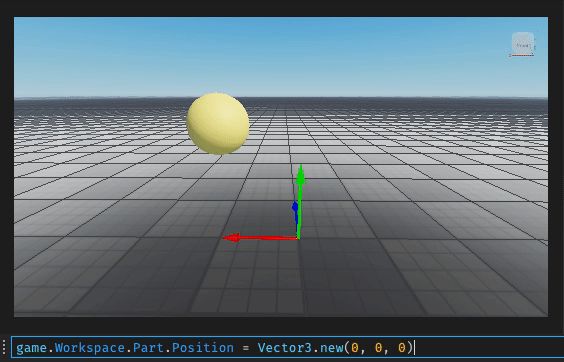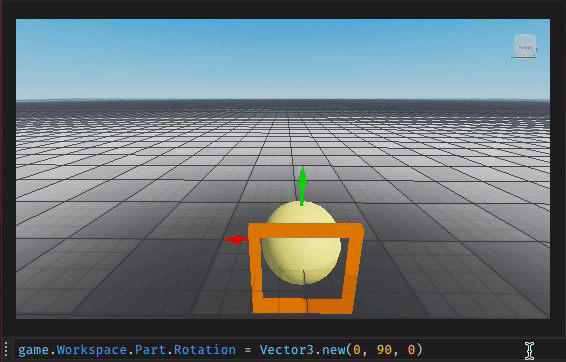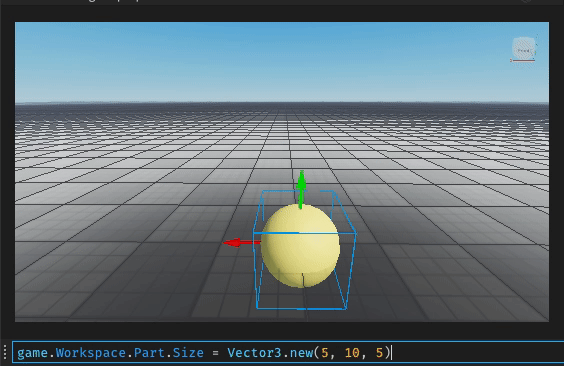## Roblox Vector3

In Roblox, a Vector3 defines a Vector with three components. We use it for setting the position, rotation, and size of elements.

For example, a Part's Position property is a Vector3 that sets its center position.game.Workspace.Part.Position = Vector3.new(0, 0, 0)


The Rotation property describes the amount of rotation along each axis in degrees.game.Workspace.Part.Rotation = Vector3.new(0, 90, 0)


The Size property describes the size of each side of a rectangular prism.game.Workspace.Part.Size = Vector3.new(0)


print(Vector3.new(1, 1, 1) + Vector3.new(2, 2, 2)) -- 3, 3 ,3


We can perform Vector Subtraction by subtracting two Vector3 instances.

print(Vector3.new(1, 1, 1) - Vector3.new(1, 1, 1)) -- 0, 0, 0


We can scale a vector by multiplying it by a scalar (Vector Scaling).

print(Vector3.new(1, 1, 1) * 2) -- 2, 2, 2


The Vector Magnitude is available via the Magnitude property. It's equivalent to the function:

function vectorMagnitude(vector)
return math.sqrt(
vector.X * vector.X +
vector.Y * vector.Y +
vector.Z * vector.Z
)
end

print(vectorMagnitude(game.Workspace.Part.Position))
print(game.Workspace.Part.Position.Magnitude)

--- 3.4641016151378
--- 3.4641015529633


Note: there is a slight difference due to the imprecision of floating-point numbers.

A use case for this is to calculate the distance between 2 vectors by subtracting them and calculating the magnitude of the returned vector.

The Unit property returns a Unit Vector, which is a new vector with a Magnitude of 1 in the direction of the original vector

Equivalent to:

function vectorUnit(vector)
return Vector3.new(
vector.X / vector.Magnitude,
vector.Y / vector.Magnitude,
vector.Z / vector.Magnitude
)
end
print(vectorUnit(game.Workspace.Part.Position))
print(game.Workspace.Part.Position.Unit)

---  0.57735025882721, 0.57735025882721, 0.57735025882721
---  0.57735025882721, 0.57735025882721, 0.57735025882721


We can perform a Linear Interpolation between 2 vectors using lerp. Helpful in placing things in between 2 parts.

Lastly, Dot performs the Dot Product between 2 vectors. Cross returns the Cross Product between 2 vectors.

Roblox (2021)

#### References

Roblox. Vector3. August 2021. URL: https://developer.roblox.com/en-us/api-reference/datatype/Vector3.